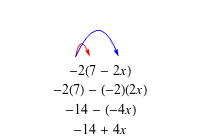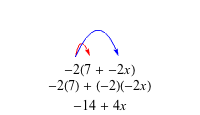Study Guide

# Distributive Property

## Distributive Property

This one is insanely important when working with algebraic expressions. The distributive property basically says this: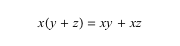and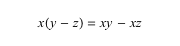However, the distributive property does not work when the variables inside the parentheses are being multiplied or divided.andLet's go through an example very carefully: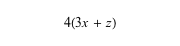By applying the distributive property, we can multiply each term inside the parentheses by 4. This is called "distributing."Since 12x and 4 are not like terms, this is as far as we can go with the problem.

What about subtraction? Let's look at a subtraction problem using two different methods.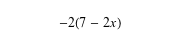Method 1
Leave as Subtraction
Method 2